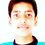Prove the following inequality

$\dfrac{a}{b+2c+3d} + \dfrac{b}{c+2d+3a} + \dfrac{c}{d+2a+3b} + \dfrac{d}{a+2b+3c} > \dfrac{2}{3}$

If a,b,c,d are distinct positive reals, prove the above inequality.Note by Raushan Sharma
3 years, 9 months ago

This discussion board is a place to discuss our Daily Challenges and the math and science related to those challenges. Explanations are more than just a solution — they should explain the steps and thinking strategies that you used to obtain the solution. Comments should further the discussion of math and science.

When posting on Brilliant:

• Use the emojis to react to an explanation, whether you're congratulating a job well done , or just really confused .
• Ask specific questions about the challenge or the steps in somebody's explanation. Well-posed questions can add a lot to the discussion, but posting "I don't understand!" doesn't help anyone.
• Try to contribute something new to the discussion, whether it is an extension, generalization or other idea related to the challenge.
• Stay on topic — we're all here to learn more about math and science, not to hear about your favorite get-rich-quick scheme or current world events.

MarkdownAppears as
*italics* or _italics_ italics
**bold** or __bold__ bold

- bulleted
- list

• bulleted
• list

1. numbered
2. list

1. numbered
2. list
Note: you must add a full line of space before and after lists for them to show up correctly
paragraph 1

paragraph 2

paragraph 1

paragraph 2

> This is a quote
This is a quote
# I indented these lines
# 4 spaces, and now they show
# up as a code block.

print "hello world"
# I indented these lines
# 4 spaces, and now they show
# up as a code block.

print "hello world"
MathAppears as
Remember to wrap math in $$...$$ or $...$ to ensure proper formatting.
2 \times 3 $2 \times 3$
2^{34} $2^{34}$
a_{i-1} $a_{i-1}$
\frac{2}{3} $\frac{2}{3}$
\sqrt{2} $\sqrt{2}$
\sum_{i=1}^3 $\sum_{i=1}^3$
\sin \theta $\sin \theta$
\boxed{123} $\boxed{123}$

Sort by:

Quite easy cyclic sum(a^2/(ab+2ac+3ad)) and apply Tittu's lemma

- 2 months, 2 weeks ago

Have u given the inmo mock test of fiitjee yesterday

- 3 years, 9 months ago

No, one of my friends gave that, and yeah, it's a question I gave from there only... Did you give that test?? How was it?

- 3 years, 9 months ago

Yes I gave the test and it was really difficult....this was the only question I could completely solve....I would post the complete paper soon.

- 3 years, 9 months ago

Ya, I have the complete paper, but can you give the solution to this inequality, I mean how you solved, I was trying it with Tittu's Lemma, but couldn't complete

- 3 years, 9 months ago

Use titu lemma and then cauchy schwarz on $sqrt (a), sqrt (b), sqrt (c), sqrt (d) and 1,1,1,1$ it would give the direct result

- 3 years, 9 months ago

Oh, yeah, I did it today, after I commented that. It was quite easy. Actually first I was not expanding $(a+b+c+d)^2$. I first applied Tittu's lemma and then AM-GM

- 3 years, 9 months ago

Can you please post the complete solution including explanation for this question??

- 3 years, 7 months ago

Yes, I can, but for that can you please tell me, how can we add an image in the comment??

- 3 years, 7 months ago

Sorry. I don't know how can we upload images in comment. I think we can't upload images in comment but and upload it in solutions for given questions. Can you please write the whole solution?

- 3 years, 7 months ago